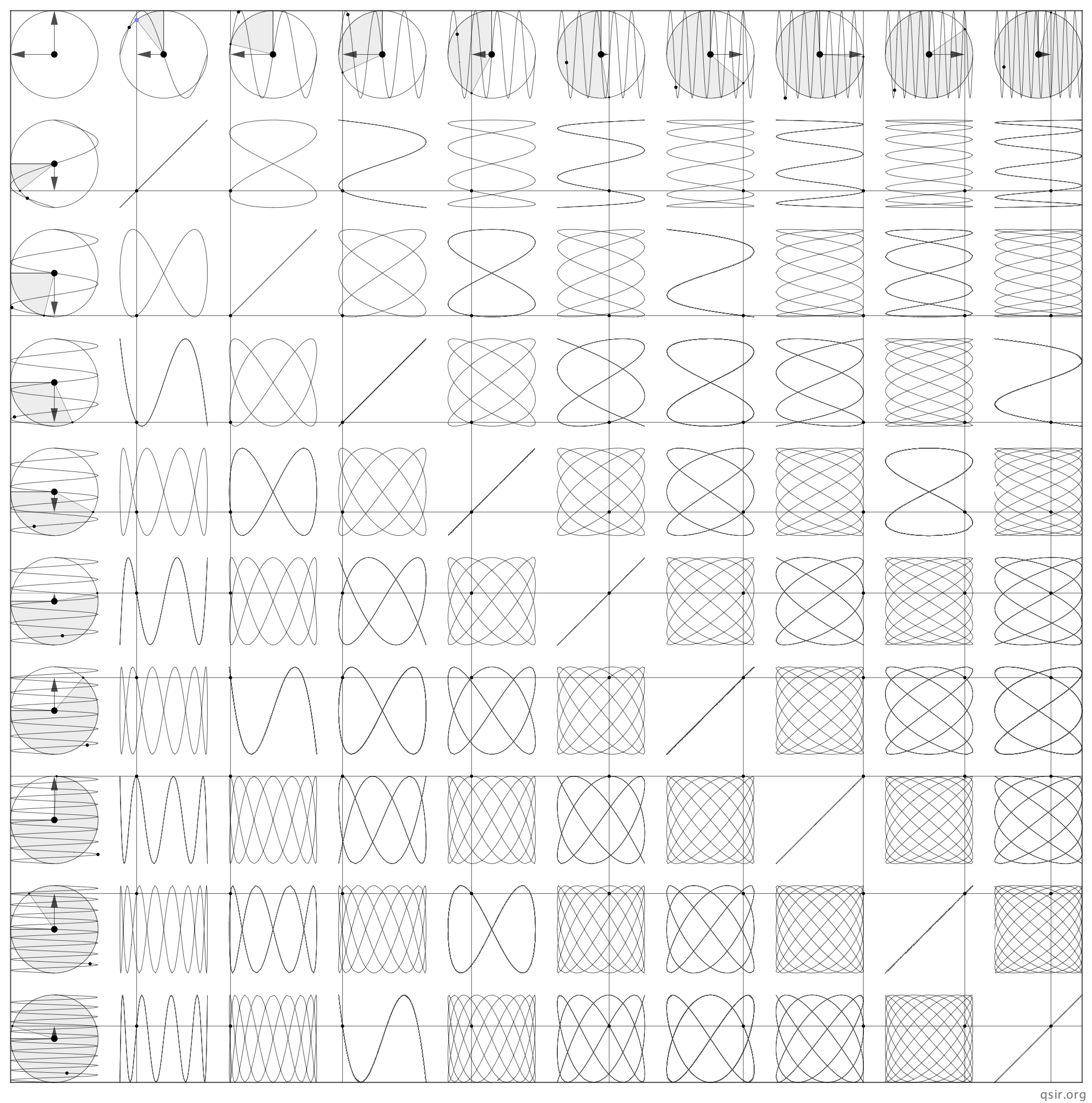## 振动不息传波不止~~~

@qiusir:这两天讲机械振动和机械波，课堂上直接构造了模型，演示了没差异的单调和依次的波动，顺带演示了下视觉暂留，学生似乎有点热情，新疆部的两位年轻老师兼球友坚持跟着听课。过两天讲讲振动叠加和纵波，希望学生不仅会解题，还能独自构造甚至探究一下...@qiusir:关于简谐波，纵波的疏部、密部相当于横波的平衡位置，而不是和波峰、波谷对应；现实的水波比较复杂，除了随波不逐流，可以把“摇曳”看成是既有水平方向又有竖直方向的振动叠加…… ​​​​
@qiusir:机械横波的干涉~~~@qiusir:演示波的传播和叠加的过程中分段函数发挥作用，同时加深了波动方程的理解，至于GeoGebra曲面的功能用到3D曲线的旋转，但效果不一样。而对于颜色的跟踪，特别是淡化效果，GSP还是很便捷，很好奇这么好的小软件咋停更了呢...
@qiusir:才注意到还有个曲面的功能，简单构造了下波的干涉...@qiusir:振动不息传波不止~~~
@Richard Feynman:Who dares to teach must never cease to learn.
@qiusir:构造了个立体的干涉图样，我学生似乎也在熬夜构造波动的模型~~~@qiusir:有留言说我最近波涛汹涌，再来一波。关于纵波干涉的模型花了我一点时间，甚至开车的时候都浸入其中~~~ ​​​​10

## 回旋加速器|cyclotron1919年卢瑟福(E.Rutherford)用天然放射源中$\alpha$ 粒子（即氦核）作为“炮弹”轰击金属箔的“靶”(atomic target)，实现了人类科学史上第一次人工核反应，发现原子核本身有结构，人们不断寻求更高能量的粒子来作为“炮弹”。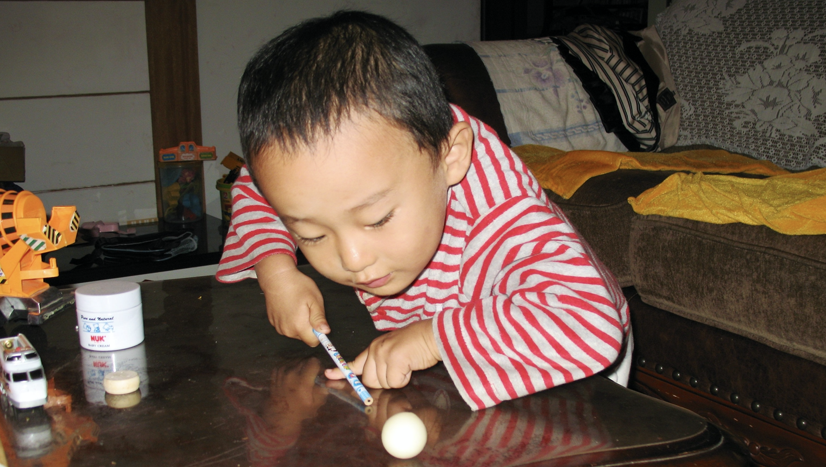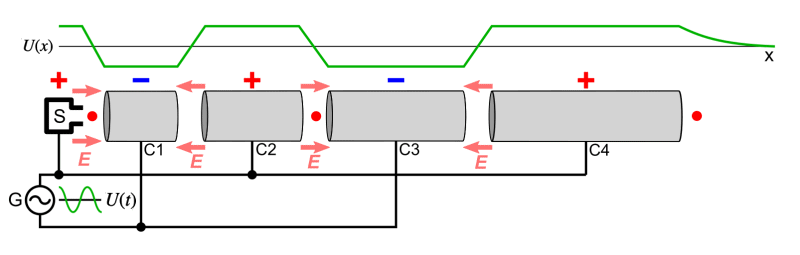via[?]按照表达习惯修改了U代表电压等细节

（为简化问题，此文把窄缝处的电场都等效为匀强电场，加速过程当成匀变速运动）
eg0:加速缝隙很小，且认为质子在每个漂移管内运行时间为半个周期。以及电压$f=1\times10^7Hz$$v_{c_1}=8\times10^6m/s$,$v_{c_4}=1\times10^7m/s$，质子比荷$\frac{q}{m}=1\times10^8C/kg$。求漂移管C1的长度和相邻两管间的加速电压...
(0.4m $6\times10^4V$)0、估算质子在1T磁场中偏转的频率和动能为1Mev的质子的速度为光速的多少倍
$f=\frac{qB}{2\pi m}$ $f=\frac{1.6\times10^{-19}C\times1T}{2\pi\times1.67\times10^{-27}kg}$ 估算约$10^7Hz$$E_k=\frac{1}{2}mv^2$,$\frac{1}{2}1.67\times10^{-27}v^2=1.6\times10^{-19}\times10^6$粗略估算约光速的0.05倍
1、带电粒子能获得的最大速度$v_{m}$

2、相邻轨道半径的比$\frac{r_{n+1}}{r_{n}}$
$nqU=\frac{1}{2}m{v_n}^2$$r_{n}=\frac{mv_{n}}{qB}$，得$r_{n}=\sqrt{\frac{2nmU}{qB^2}}$
$\frac{r_{n+1}}{r_{n}}=\sqrt{\frac{n+1}{n}}$，大概能理解轨道越来越密集...3、带点粒子被电场加速的次数$n$

4、带电粒子在磁场中运行的总时间$t_{B}$

$t_{B}=\frac{\pi{B}R^{2}}{2U}$

5、带电粒子在电场中运行的总时间$t_{E}$

$t_{E}=\frac{BRd}{U}$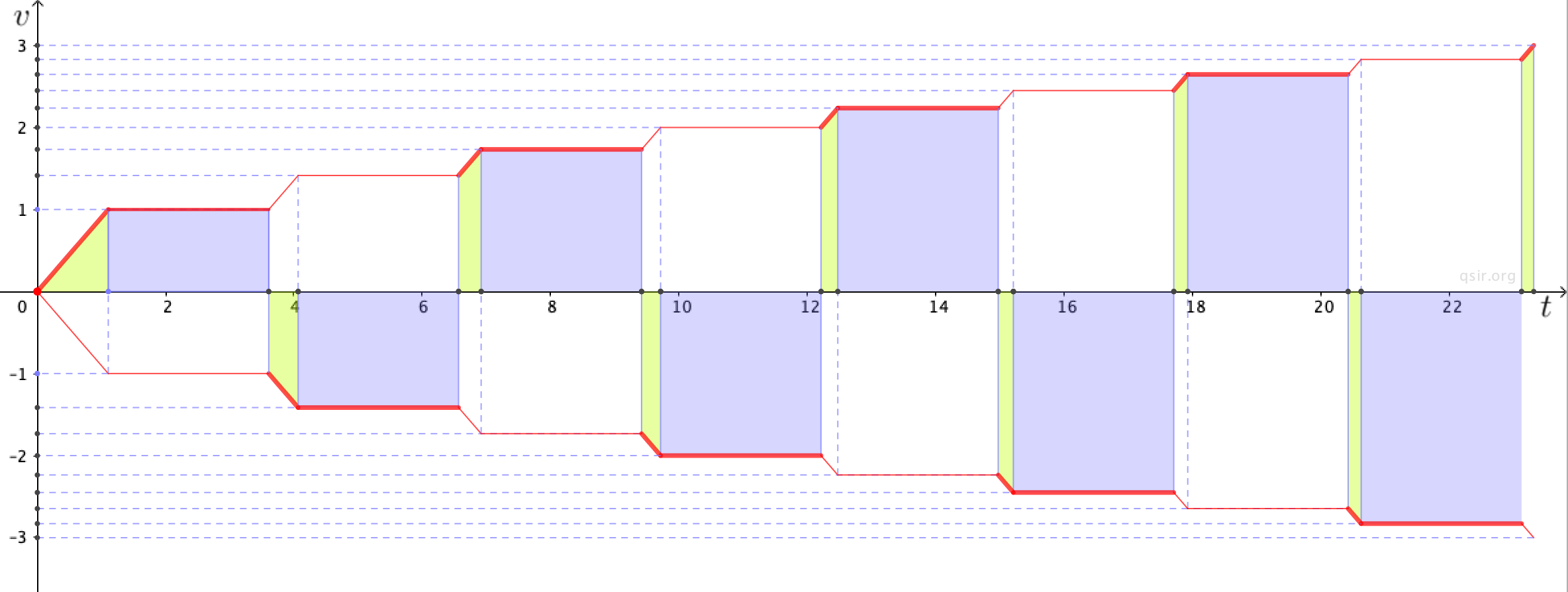6、带电粒子在磁场中运动时间与在电场中运动时间的比较$\frac{t_{B}}{t_{E}}$

（通常一个粒子在回旋加速器中心飞行到提取装置的总时间约为几个ms）
7、对不同粒子的加速问题$_{1}^{1}H$$_{1}^{2}H$$_{1}^{3}H$$_{2}^{4}He$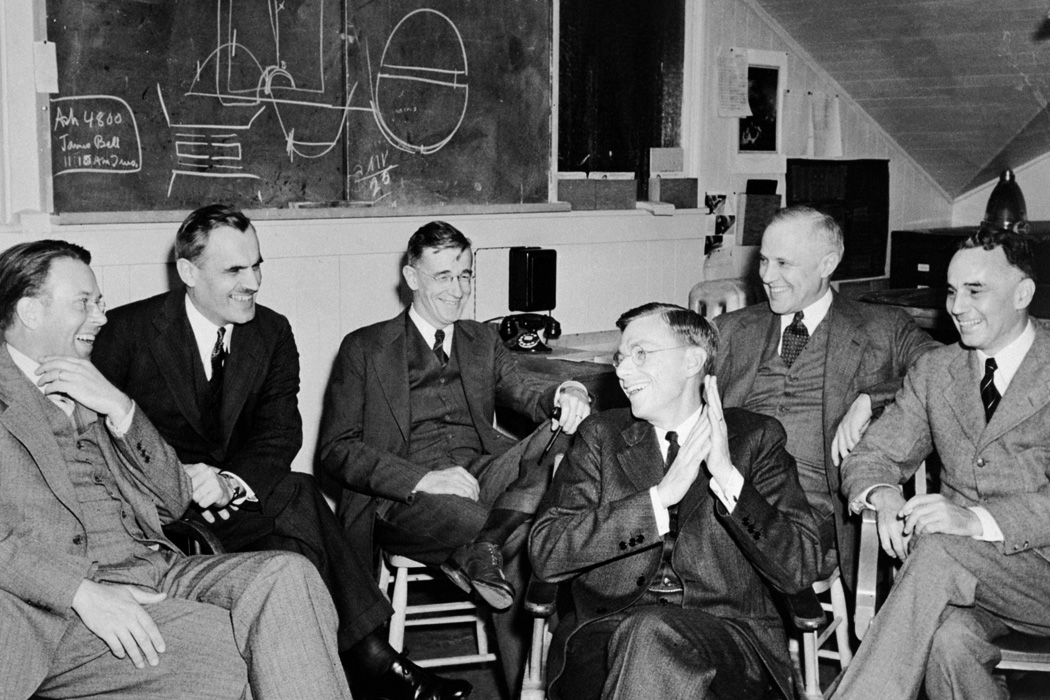eg1:计算第一台回旋加速器能使质子获得的最大动能？（半径0.1m，B=1T，m=1.67×${10}^{-27}T$）（结合前面估算具有1MeV动能的质子速度数值，加速电子会怎样）（直径只有12厘米，可以拿在手里，加速粒子能量可达到0.8MeV）

eg2:某回旋加速器交变电压频率$f$，可将原来静止的$\alpha$粒子$_{2}^{4}He$加速到最大速率$v$，使它获得的最大动能为$E_k$，加速次数$n$。忽略带电粒子的初速度、所受重力、以及通过狭缝的时间，且不考虑相对论效应，交流电频率调至$2f$，则用该回旋加速器加速静止的质子$_{1}^{1}H$，能使其获得的最大速度为$2v$、最大动能$E_k$，被加速的次数$2n$

eg3:两个相同的回旋加速器分别接在加速电压为$U_1$$U_2$的高频电源上,且$U_1>U_2$。有两个相同的带电粒子分别在这两个加速器中运动，设两个粒子在加速器中运动的时间分别为$t_1$$t_2$，获得的最大动能分别为$E_{k1}$$E_{k2}$，比较他们的大小。

eg4:实际使用中，磁感应强度和加速电场频率都有最大值的限制。若某一加速器磁感应强度和加速电场频率的最大值分别为$B_m$$f_m$，则带电粒子粒子能获得的最大动能$E_{km}$
$f=\frac{qB}{2\pi{m}}$$f_B=\frac{qB_m}{2\pi{m}}$，如果$f_m，最大动能由$B_m$决定，否则由$f_m$决定。

"Lawrence will always be remembered as the inventor of the cyclotron, but more importantly, he should be remembered as the inventor of the modern way of doing science."
—Luis Alvarez, winner of the 1968 Nobel Prize for Physics

1931年，劳伦斯和他的学生们建成了第一台回旋加速器，直径只有12厘米，加速粒子能量可达到0.8MeV。后来，在劳伦斯的领导下，在美国建成了一系列不同的回旋加速器。回旋加速器的Dee盒内要有真空的条件，交流电压的频率$10^7$
E.O.Lawrence was the "father of big science", the first to advance the idea of doing research with multidisciplinary teams of scientists and engineers.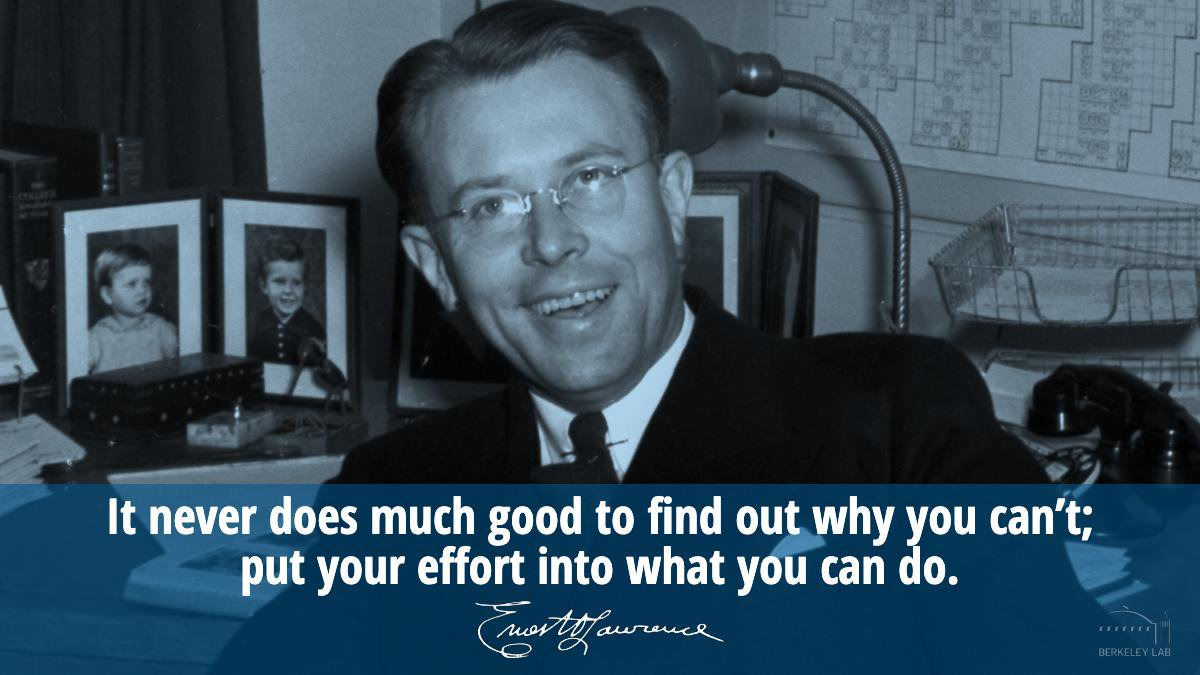“It never does much good to find out why you can’t; put your effort into what you can do.”
— Ernest Lawrence

B(magnetic flux density)

H(magnetic field strength)

who what where when why how...

$\mathfrak{qiusir@qiutopia}$

1、课上讲解和交流的内容是从准备的材料中提炼出来的，或说是从某一条线索推进的。主要是看核心的问题是否凸显了，然后才是问题是否清楚了，后面才是相关问题解决和熟练的练习...
2、关于引入，通过臧同学专注的击球的照片，设想未来的他，是专业的运动员？在少年班读书的他更可能成为轰击原子核甚至更小微粒的科学家。科学家身后黑板上的图和今天的课题很相近，甚至MIT卢因教授的板书也和今天课题呼应，学生大都GET了这个点，至于我特地放了瓶可乐，由于时间紧，没怎么说...从物理的角度有两种极限，一是大的极限，比如银河比如宇宙。另一种极限是小的极限，原子核，核子，夸克等等，而回到臧同学的未来，加速器不仅在探究物质机构，在医疗在生物等等方面都有应用。他的未来，无论是向着大物体还是小物体发展，都是“全方位的无限”...
3、看到外国语学校的同事来，有新疆部、理科部和少儿部的来，当然更多是高中部的，有几位别的学科老师也来了，感谢捧场...
4、杨专家评课，提到首席教师...教化学出身的他老人家还特地先学习了加速器相关知识。提到不仅是知识传授，还强调知识探究方法，引用了大脑不是容器是火把的名言。说到板书很科学，黑板白板相结合，颜色的信息凸显。不仅关心孩子的问题解决，还重视学生问题的提出...个人素质好，整合能力强。幽默，“生活中的下坡路不要悲观，动能会增加...”让他印象深刻。
5、张组长评课，提到上课的节奏感，不紧不慢，张弛有度；即便是学生没有明白也不放弃交流；课堂内外，科学素养；小孩的照片引入，PPT耳目一新，重视与学生互动...
6、杨老师评课，她很有热情，更是慷慨。很抬举我说了不少好话哈哈。提到我选了这个课题，就如我骄傲又任性哈哈，提到创新、精确的构图，提到艺术、人文的气息，颜色的信息和识别，做到了艺术美和科学美的叠加。从直线加速器到回旋加速器的呼应和递进。课后她特地拷贝了几张图...外国语的葛老师也来听课了，说重视思维培养，重视物理研究...·高中部第258期每周一课[?]
7、感谢参与班级高二3班同学们的支持，感谢吴杰和求师得Laura、May拍照...感谢于鉴轩和石越洋两位同学的软文...另，投影用的是下面这张图片：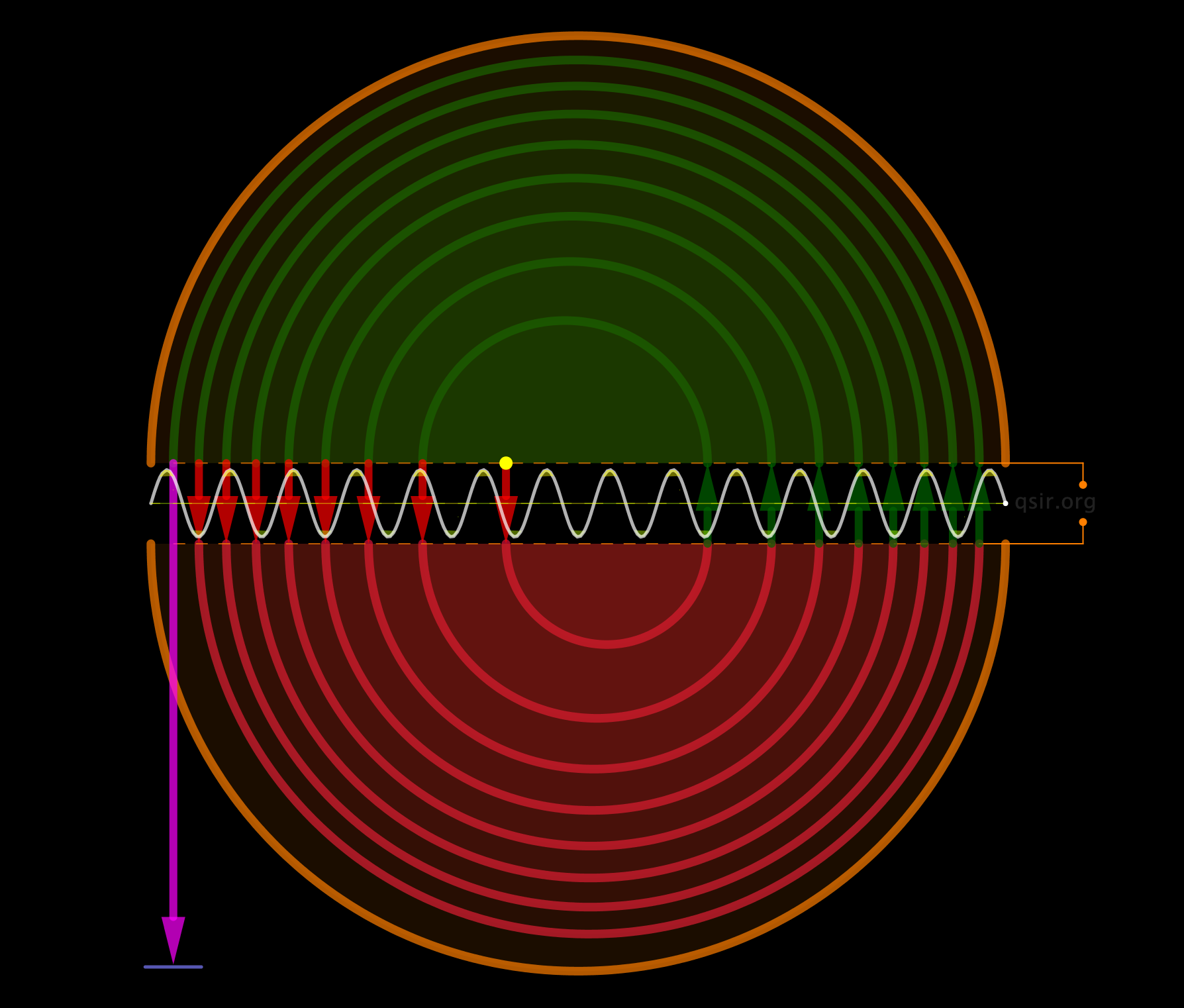P.S.多日后，食堂里遇见学校的专家，特别提醒给我留言了，看了下士关于ABCD中D的读音，应该读di，不要读de。还特地问了下学生们，大家都接受为了方便区分选择题B\D而读de，包括英语老师有的也读de的。我记得大学时，公共课老师读Boy\Dog，有学生还告诉我，北京附近有还读dei，哈哈...姥专家不仅给我留了言，还写了长文。其实吧，关于矢量还是向量的说法，钱三强都让大家自己选择吧...

04

## 李萨茹图的九九表